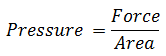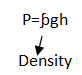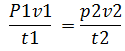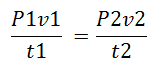Monday , June 24 2019

Pressure

Pressure is defined as the force acting per unit area.SI unit: Pascal (P) or Nm-2

Pressure In Liquids:

Pressure in liquids is given by the formula:

Pressure = density × gravity × heightPressure increases with the depth and density of the liquid.

While calculating pressure in liquids height should be always measured from the bottom.

Pressure In Gases:

Pressure in gases is due to the bombardment of molecules on walls of container and it can be measured by manometers.

Barometer:

It is an instrument for measuring atmospheric pressure. There are two types of barometers mercury barometer and water barometer

Boyle’s  Law:

Boyle’s law states that the pressure exerted by gas is inversely proportional to the volume provided the temperature remains constant.

Therefore:P1v1 = p2v2

Whenever temperature increases the volume increasesMass Weight And Density

Mass: Mass is the measure of amount of substance in a body. (Irrespective of its …

1.2.3.